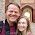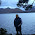Thursday, October 11, 2012

Stata - Write your own "fast" bootstrap

* Imagine we would like to bootstrap the standard errors of a command using a bootstrap routine.

* I created a previous post demonstrating how to write a bootstrap command.  This is a similar post however the bootstrap is much faster than the previous one.

* First let's generate some data.

clear
set obs 1000
gen x1 = rnormal()
gen x2 = 2*rnormal()
gen u = 5*rnormal()

gen y = 5 + x1 + x2 + u

local dependent_var x1 x2

local command_bs reg y `dependent_var'

* First let's see how the base command works directly.
`command_bs'

* As a matter of comparison this is the built in bootstrap command.
bs, rep(100): `command_bs'

* The following code is yet another user written bootstrap alternative.

* I wrote this

* Specify the number of bootstrap iterations
local bs = 100

* Save the number of observations to be drawn
local N_obs = _N

* Number of terms plus one for the constant of coefficients to be saved
local ncol = wordcount("`dependent_var'")+1

mata theta = J(`bs',`ncol',0)
forv i = 1(1)`bs' {
* Preserve the initial form of the data
preserve

* Draw the indicators of the resample
mata draw = ceil(runiform(`N_obs',1):*`N_obs')

* Create an empty matrix to hold the number of items to expand
mata: expander = J(`N_obs',1, 0)

* Count the number of items per observation to generate.
qui mata: for (i=1 ; i <= `N_obs'; i++) expander[i]=sum(draw:==i) ; "draws complete"

* Pull the expander value into stata
getmata expander=expander

* Drop unnessessary data
qui drop if expander == 0

* Expand data, expand==1 does nothing
qui expand expander

* Run a regression
qui `command_bs'

* Send to mata the matrix of results
mata theta[`i',] = st_matrix("e(b)")

* Configure the visual display
di _c "."
if (int(`i'/10)==`i'/10) di _c "|"
if (int(`i'/50)==`i'/50) di " " `i'

restore
}

* The estimates of the coefficients have been saved into a theta matrix.
mata theta

* Now let's calculate the standard deviations.
mata bs_var = variance(theta)
mata bs_var
mata bs_se = diag(bs_var):^.5
mata bs_se

1.this cannot work as you mix the referencing of locals in stata and mata context. In Mata `local' does not work. you have to use st_local("local").

1.Ah, but it does work. Try it out :D

The reason I believe is because I have not yet switched to the mata console and am instead using mata as a prefix. Perhaps this is not the best way to do this but it works.

2.Hi.. How can I bootstrap a test linear hypotheses after estimation

thierry

1.Hi Thierry,

Check out:
http://www.econometricsbysimulation.com/2012/07/write-your-own-bootstrap-command.html

That might give you what you are looking for though I am pretty sure unfortunately you have a more fundamental problem with the theory of the analysis you are trying to perform (judging from your linkedin help requests).

Francis

3.Hello,

I have started the same thread in "Applied Statistics" but I got no answer so maybe this sections is more appropriate.

I'm trying to perform a panel regression using STATA. I have an unbalanced panel containing 313 companies over 15 years, 3005 observations. I am planning to use the xtreg, fe or xtreg, re commands and I'm familiar with Hausman test, tests for autocorr, heteroskedasticty and how to perform robust regressions etc. However I have a few problems before I get going.

My questions are the following

1) Does the OLS regression assumption of Normally distributed residuals apply to Panel Regressions?

2) If it does, should I check for e to be normally distributed (not u)?

3) If I need normality should it be enough to only transform and normalize my dependent variable? (I've read this on statalist)

4) I've tried to transform my dependent variable (and independent variables) using the STATA command "ladder" though none of the options (cubic, square, square root, log, 1/square root, inverse, 1/square, 1/cubic) have been successfull. All my variables are ratios and exhibit kurtosis and skewness even after transformation. I have checked for normality using Shapiro Wilk, Shapiro- Francia, Skewness Kurtosis tests.

5) As a last resort, I have tried a Boxcox transformation but I am not sure if I am doing it correctly. If I am not mistaken it should be capable of normalizing any data. Is this true? If so, can someone please explain in layman's terms how to perform it and normalize a variable in Stata and also interpret the results from Boxcox.

6) If I am not able to transform my variables so that they are normally distributed (and get normally distributed residuals) can I perform a panel data regression in STATA based on any other distributions? If so, how and what distribution? Can you provide any links?

I know I've asked alot of questions and some may not make sense but I am beginner in econometrics and I would REALLY appreciate any help as I am lost at sea. If you would like to take a look at my data and/or descriptive stats for my variables I would be happy to send it.

I attached a picture to give you an Idea of how my variables are distributed as compared a to normal distribution. The top three are dependent variables ROA ROE M-to-B. I will perfrom 3 seperate regressions (one with each as dependent variable).

Thank you,

1.Hi Abrahim,

This is quite a question set. Try posting this same question set perhaps on CrossValidated (http://stats.stackexchange.com/). Though to give you a short response that may be of guidance: 1. yes (standard OLS assumes the same thing no matter the data structure) 2. not sure what you mean 3. sounds like a bad idea to me 4. normality is really generally only an assumption important for the sake of efficiency 5. um, forget normalization :) 6. OLS does not require normality in order to imply consistency.

Good luck!